Indexing of Trigonal and Hexagonal Systems
- Practical Electron Microscopy and Database -
- An Online Book -
Microanalysis | EM Book                                                                                   https://www.globalsino.com/EM/

This book (Practical Electron Microscopy and Database) is a reference for TEM and SEM students, operators, engineers, technicians, managers, and researchers.
=================================================================================

For hexagonal lattices, there are normally three choices of unit cell as shown in Figure 3091a:
i) Miller indices. Figure 3091a (a) shows the primitive, smallest hexagonal unit cell. However, this does not reveal the hexagonal symmetry of the lattice and is inconvenient. For instance, all the faces parallel to the z-axis are in the same form.
ii) Miller-Bravais indices. In Figure 3091a (b), a fourth axis (the u-axis) is inserted at 120° to both the x- and y-axes with so-called Miller-Bravais axes x, y, u, z, resulting in four numbers (hkil). Note that the sum of the first three numbers is zero, that is, h+k+i = 0, and thus i = -(h+k). To distinguish similar planes rotated by 120° in HCP, this Miller-Bravais indexing is more important than Miller indexing.
iii) Figure 3091a (c) shows orthohexagonal cell. Similar to i), this does not reveal the hexagonal symmetry of the lattice. However, this base-centered cell has the advantage that the axes are orthogonal and it is especially helpful to show small distortion of similar structures, e.g. the symmetry change from hexagonal to orthorhombic lattices.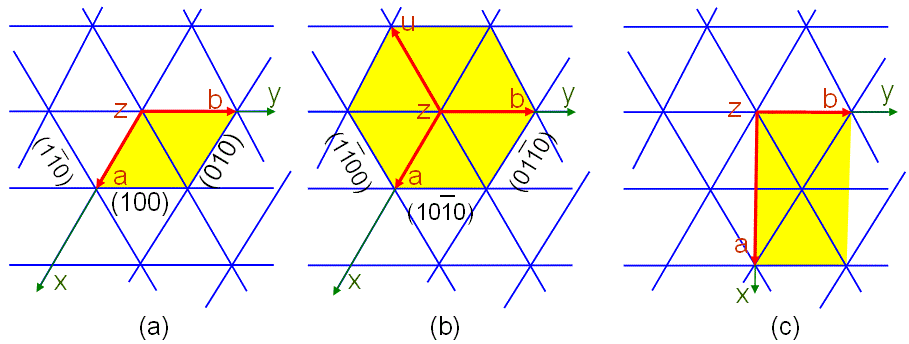Figure 3091a. Hexagonal net of the hexagonal P lattice: (a) Primitive hexagonal unit cell with six prism faces indexed {hkl}, (a) Hexagonal (four-index) unit cell with six prism faces indexed {hkil} and (c) Orthohexagonal unit cell. The z-axis points out of the plane of the page.

The three-axis notation in Figure 3091a (a) can be converted to the four-axis notation in Figure 3091a (b) with the equations below: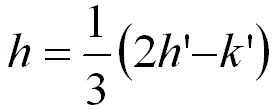-------------------------------- [3091a]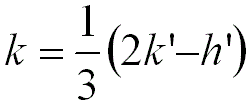-------------------------------- [3091b]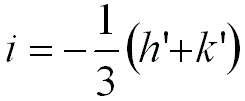-------------------------------- [3091c]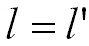-------------------------------- [3091d]
where,
h', k', and l' -- The indices in the three-axis system.
h, k, i, l -- The indices in the four-axis system.

For instance,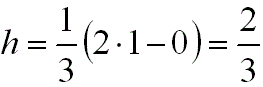-------------------------------- [3091e]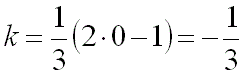-------------------------------- [3091f]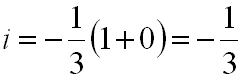-------------------------------- [3091g]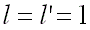-------------------------------- [3091h]
where,
h', k', and l' -- 1, 0, and 1, respectively.
Because the obtained h, k, i, and l contain fractions, their values need to be reduced to lowest integers. Therefore, the direction <2 -1 -1 3> in the four-axis system is equal to the direction <1 0 1> in the three-axis system.

Similarly, the converting of hexagonal zone axes between Miller notation [U V W] and Miller-Bravais notation [u v t w] can be given by:
U = u - t --------------------------------- [3091i]
V = v - t --------------------------------- [3091j]
W = w --------------------------------- [3091k]
And,
u = (2U - V)/3 --------------------------------- [3091l]
v = (2V - U)/3 --------------------------------- [3091m]
t = - (u + v) = - (U + V)/3 --------------------------------- [3091n]
w = W --------------------------------- [3091o]

For your convenience, the four-axis notations can be converted to the three-axis notations with the excel file, vice versa.

Figure 3091b shows different crystal planes in hexagonal and trigonal crystal structures with four-index. The a-planes are given by the {11-20} facets, the c-planes by the {0002} facets, the m-planes by the {1-100} facets, and the r-planes by the {1-102} facets of the hexagonal unit cell.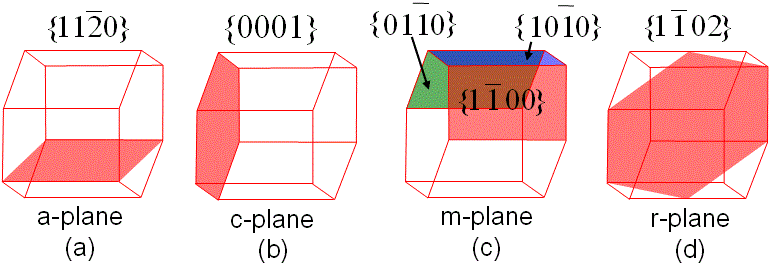Figure 3091b. Different planes in the hexagonal and trigonal crystal lattices.

Now, we can figure out the Miller-Bravais notation for vector A in Figure 3091c:
Step i) Project the vector onto the basal plane, which is labeled as A’ between a and b in Figure 3091c.
Step ii) Obtain the direction of A' vector: Because A' = (a + b), its direction will be [1 1 0] in coordinates of [a b c]. Here, c-intercept is 0.
Step iii) In Miller-Bravais index (4-index), vector A' will be (1/3) [1 1 -2 0].
Step iv) In 4-index notation, then c = [0 0 0 1].
Step v) Then, we can get the reduced A = [1 1 -2 3].Figure 3091c. Example of extractions of Miller-Bravais notations.

For hexagonal structures, the lattice spacing (d-spacing) can be given by, (You can download the excel file for your own calculations)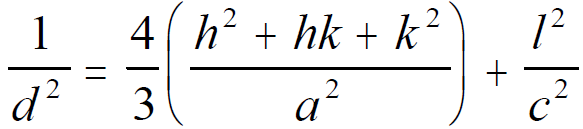--------------------------------- [3091p]
where,
a and c -- The lattice constants.
h, k, and l -- The Miller indices.

=================================================================================
The book author (Yougui Liao) welcomes your comments, suggestions, and corrections, please click here for submission. If you let book author know once you have cited this book, the brief information of your publication will appear on the “Times Cited” page.